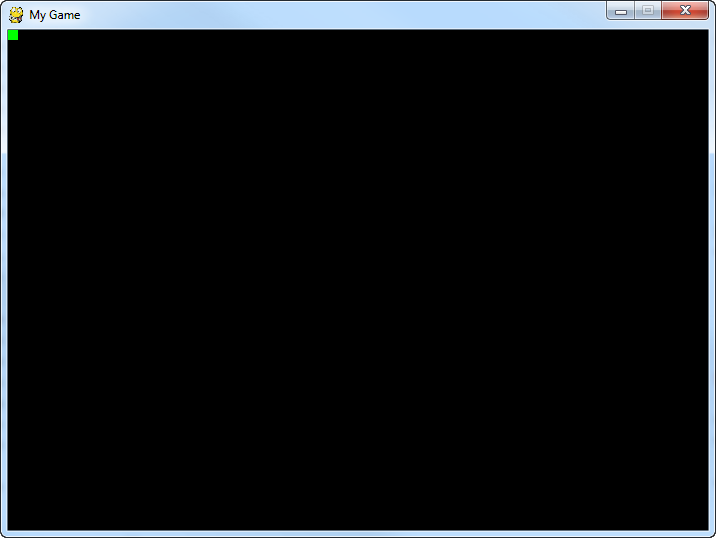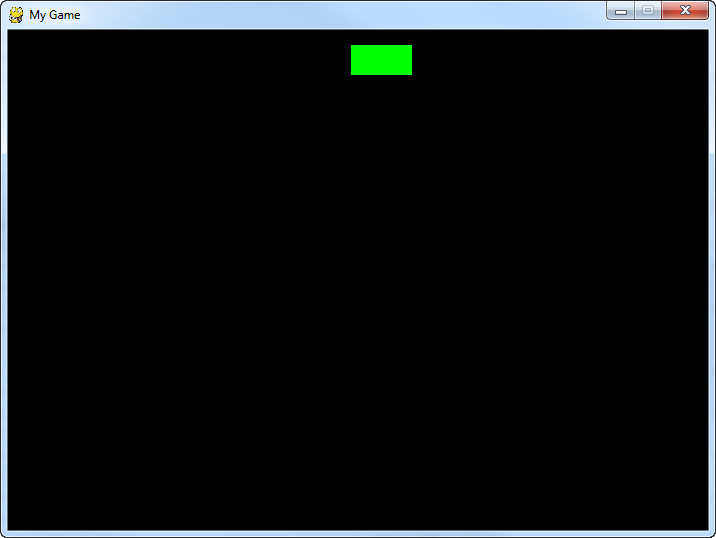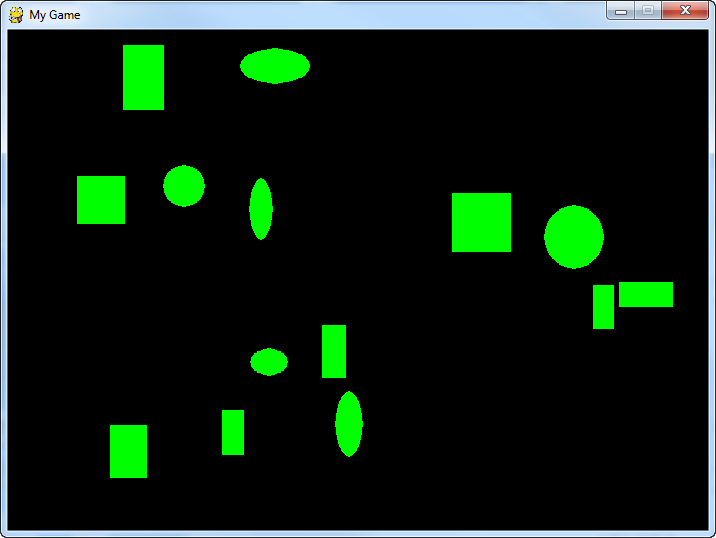﻿﻿ Программирование аркадных игр и обучение информатике

# Программирование аркадных игри обучение информатике

 < Оглавление >

# Lab 12: Classes and Graphics

Graphics provide an excellent opportunity to use classes. Each graphic object can be represented by an object. Each type of graphic object can be represented by a class. An object's location, speed, and color can be stored in attributes.

## 12.1 Instructions

1. Start a new program with:
2. Right after the default colors are defined in the example program, create a class called Rectangle.
• Add x and y attributes, which will be used for storing the object's position.
• Create a draw method. Have the method create a green 10x10 rectangle at the location stored in x and y. Don't forget to use self. before the variables. The method will need to take in a reference to screen so that the pygame.draw.rect function can draw the rectangle to the correct screen.
3. Before the program loop, create a variable called my_object and set it equal to a new instance of Rectangle.
4. Inside the main program loop, call my_object's draw() method.
5. Checkpoint: Make sure your program works, and the output looks like Figure 33.3.Figure 33.3: Rectangle in top left corner
6. Right after the program creates the instance of Rectangle, set the x and y values to something new, like 100, 200. Run the program again to make sure it works and the rectangle moves to the new coordinates.
7. Add attributes to the class for height and width. Draw the rectangle using these new attributes. Run the program and make sure it works.
8. Get the object to move:
• Add attributes for change_x and change_y.
• Create a new method called move(), that adjusts x and y based on change_x and change_y. (Note that the move method will not need screen as a parameter because it doesn't draw anything to the screen.)
• Set my_object's change_x and change_y to values, like 2 and 2.
• Call the move() method in the main program loop.
• Test to make sure the object moves.
9. Randomize the object
• Import the random library
• Set the x location to a random number between 0 and 700. You can do this in the loop where you create the object, or it can be done in the __init__ method.
• Set the y location to a random number between 0 and 500.
• Set the height and width to a random number between 20 and 70.
• Set the change_x and change_y to random numbers between -3 and 3.
• Test and make sure it looks like Figure 33.4.Figure 33.4: Rectangle in random spot
10. Create and display a list of objects
• Before the code that creates the my_object, create an empty list, called my_list
• Create a for loop that loops 10 times.
• Put the code that creates my_object into the for loop
• Append my_object to my_list.
• Inside the main program loop, loop through each item of my_list.
• Call the draw and move methods for each item of the list.
• Make sure that the code calls the draw method of the element pulled out by the for loop, don't just use my_object.draw(). This is one of the most common mistakes.
11. Use inheritance
• After the Rectangle class, create a new class called Ellipse.
• Set Rectangle to be the parent class of Ellipse.
• You do NOT need to create a new ___init__, we will just inherit the parent class's method.
• Create a new draw method that draws an ellipse instead of a rectangle.
• Create a new for loop that adds 10 instances of Ellipse to my_list in addition to the 10 rectangles. (Just use two separate for loops.)
• Make sure you don't create a new list, just add them to the same my_list.
• Since both rectangles and ellipses were added to the same list, you only need one loop to go through the list and draw both. Don't make the mistake of having two loops, one for rectangles and one for ellipses. It doesn't work that way.
• Test and see if your program looks like Figure 33.6.Figure 33.6: Rectangles and ellipses
12. Make it more colorful
• Adjust the program, so that color is an attribute of Rectangle.
• Draw the rectangles and ellipses using the new color.
• In the for loops, set the shapes to random colors. Remember, colors are specified by three numbers in a list, so you need a list of three random numbers (r, g, b)